Dersleri yüzünden oldukça stresli bir ruh haline sikiş hikayeleri bürünüp özel matematik dersinden önce rahatlayabilmek için amatör pornolar kendisini yatak odasına kapatan genç adam telefonundan porno resimleri açtığı porno filmini keyifle seyir ederek yatağını mobil porno okşar ruh dinlendirici olduğunu iddia ettikleri özel sex resim bir masaj salonunda çalışan genç masör hem sağlık hem de huzur sikiş için gelip masaj yaptıracak olan kadını gördüğünde porn nutku tutulur tüm gün boyu seksi lezbiyenleri sikiş dikizleyerek onları en savunmasız anlarında fotoğraflayan azılı erkek lavaboya geçerek fotoğraflara bakıp koca yarağını keyifle okşamaya başlar

GET THE APP

Analysis of Pressure Transient Tests in Naturally Fractured Reservoirs | OMICS International
Oil & Gas Research

Like us on:

# Analysis of Pressure Transient Tests in Naturally Fractured Reservoirs

Gamal Rezk M*

Gaza University, Cairo, Egypt

*Corresponding Author:
Gamal Rezk M
Assitant Professor, Gaza University
Cairo, Egypt
Tel.: +201002058903, +20227039306
E-mail: mohamedgamalrezk@gmail.com

Received Date: October 15, 2016; Accepted Date: October 19, 2016; Published Date: October 25, 2016

Citation: Gamal Rezk M (2016) Analysis of Pressure Transient Tests in Naturally Fractured Reservoirs. Oil Gas Res 2: 121. doi: 10.4172/2472-0518.1000121

Copyright: © 2016 Gamal Rezk M. This is an open-access article distributed under the terms of the Creative Commons Attribution License, which permits unrestricted use, distribution, and reproduction in any medium, provided the original author and source are credited.

Visit for more related articles at Oil & Gas Research

#### Abstract

Pressure transient tests in naturally fractured reservoirs often exhibit non-uniform responses. Different techniques can be used to analyze the pressure behavior in dual porosity reservoirs in an attempt to correctly characterize reservoir properties. In this paper, the pressure transient tests in naturally fractured reservoirs were analyzed using conventional semi-log analysis, type curve matching (using commercial software) and Tiab’s direct synthesis (TDS) technique. In addition, the TDS method was applied in case of a naturally fractured formation with a vertical hydraulic fracture. These techniques were applied to a single layer naturally fractured reservoir under pseudosteady state matrix flow. By studying the unique characteristics of the different flow regimes appear on the pressure and pressure derivative curves, various reservoir characteristics can be obtained such as permeability, skin factor, and fracture properties. For naturally fractured reservoirs, a comparison between the results semi-log analysis, software matching, and TDS method is presented. In case of wellbore storage, early time flow regime can be obscured that lead to incomplete semi-log analysis. Furthermore, the type curve matching usually gives a non-uniqueness solution as it needs all the flow regimes to be observed. However, the direct synthesis method used analytical equation to calculate reservoir and well parameters without type curve matching. For naturally fractured reservoirs with a vertical fracture, the pressure behavior of wells crossed by a uniform flux and infinite conductivity fracture is analyzed using TDS technique. The different flow regimes on the pressure derivative curve were used to calculate the fracture half-length in addition to other reservoir properties. The results of different cases showed that TDS technique offers several advantages compared to semi-log analysis and type curve matching. It can be used even if some flow regimes are not observed. Direct synthesis results are accurate compared to the available core data and the software matching results.

#### Keywords

Naturally Fractured Reservoirs; Pressure Transient Analysis; Vertical Fracture; Uniform Flux Fracture; Infinite Conductivity Fracture

#### Nomenclature

B: Formation volume factor, res bbl/stb

Ct: Total compressibility, psi-1

C: Wellbore storage coefficient, bbl/psi

CA: Shape factor

Cdw: Dimensionless wellbore storage

h: Total formation thickness, ft

Kf: Bulk fracture permeability, md

p: Pressure, psi

PD: Dimensionless pressure

PwD: Dimensionless bottom-hole pressure

Pint: Initial pressure, psi

Pwf: Bottom-hole pressure, psi

P?D: Dimensionless pressure derivative

P?wD: Dimensionless bottom-hole pressure derivative

ΔP: Pressure difference, psi

qt: Flow rate, stbd

S: Skin factor

t: Test time, hr

tD: Dimensionless time

Xe: Half side of rectangle in x-axis, ft

Xf: Fracture half length, ft

ye: Half side of rectangle in y-axis, ft

#### Greek Symbols

λ: Interporosity flow parameter

ω: Dimensionless storage coefficient

μ: Viscosity, cp

φ: Porosity

#### Subscripts

b1: Beginning of first radial flow line

b2: Beginning of second radial flow line

D: Dimensionless

e: Outer boundary

e1: End of first radial flow line

f: Fracture

m: Matrix

L: Linear

o: Oil

t: Total

#### Introduction

The analysis of pressure data received during a well test in dual porosity formation has been widely used for reservoir characterization. Conventional semi-log analysis and log-log type curve methods are the early techniques used to analyze pressure transient data. However, both methods need certain criteria to give accurate results, such as; all flow regimes must be identified in the pressure and pressure derivative plot. In case some flow regimes are not identified, type curve matching will give a non-uniqueness solution and is essential trial and error, and semi-log analysis cannot be completed. Tiab  used a new method to analyze pressure transient tests, called “Direct Synthesis Technique”. This method can calculate different reservoir paramters without type curve matching by using pressure and pressure derivative loglog plots. In 1994, Tiab  extended the work to vertically fractured wells in closed system. Engler and Tiab  developed direct synthesis method to analyze pressure transient tests in dual porosity formation without using type curve matching. They used analytical and empirical correlations to calculate the naturally fractured reservoir parameters. Jalal  discussed the analytical solutions of wells in dual porosity reservoirs with a vertical fracture. The direct synthesis method offers manys advatages in analyzing pressure transient tests.

The objective of this paper is to analyze pressure transient in naturally fractured reservoirs using: conventional semi-log analysis, type curve matching (using commercial software), and Tiab’s direct synthesis method to correctly characterize the reservoir properties. These techniques were applied to naturally fractured reservoirs, with and without hydraulic (vertical) fracture.

#### Properties of Dual Porosity Formation

The Dual porosity reservoir consists of primary and secondary porosity which are the matrix and fractures. Warren and Root  defined the fractured reservoirs by two key parameters, ω and λ. These dimensionless paramters are defined as follows:

The relative storativity,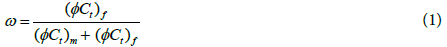The interporosity flow parameter,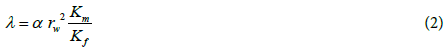Where the shape factor α, ft-2, depends on the matrix block geometry (horizontal slab or spherical matrix block). By assuming that the reservoir is infinite acting and producing a single phase, slightly compressible fluid with pseudosteady state matrix flow, the pressure solution is given by :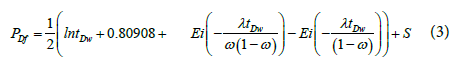Conventional semi-log analysis

Naturally fractured reservoirs give two parallel semi-log straight lines in plot of drawdown and build-up tests as shown in Figure 1.

Permeability thickness product: The permeability thickness product of the total system (actually of the fractures as the matrix permeability can be neglected) can be calculated from the slope of the initial or final straight line, m.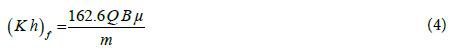1. The relative storativity ω can be calculated from the pressure difference, ΔP, between the initial and final straight lines when both of them can be identified.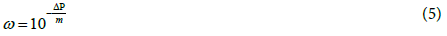2. By drawing a horizontal line through the middle of transition period to intersect with both semi-log straight lines, the times of intersection with the first and the second semi-log straight lines are donated by t1 and t2, respectively. The storativity ratio also can be determined as follows :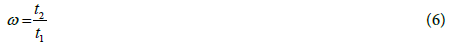3. The interporosity flow coefficient, λ, can be calculated by :

For drawdown tests: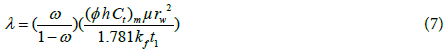or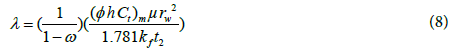For build-up tests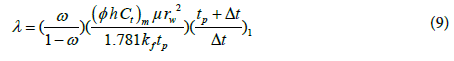or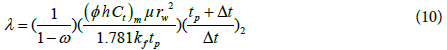Direct synthesis technique

Direct synthesis method uses a log-log plot of pressure and pressure derivative data versus time to calculate various reservoirs and well parameters. It uses the pressure derivative technique to identify reservoir heterogeneities. In this method, the values of the slopes, intersection points, and beginning and ending times of various straight lines from the log-log plot can be used in exact analytical equations to calculate different parameters as it is shown in the following procedures : Infinite Acting Reservoir without Wellbore Storage

1. Fracture permeability: The fracture permeability, Kf, can be determined using early or late time infinite acting radial flow lines (only one of the two derivative segments needs to be observed)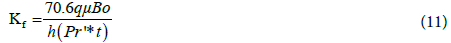2. Relative storativity: The ratio between minimum and radial pressure derivative values can be used in equation to calculate ω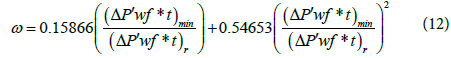The relative storativity can also be calculated by using the characteristic times as in the following equations: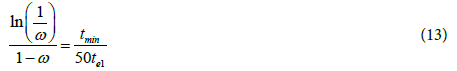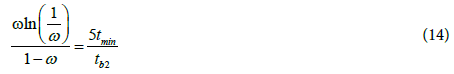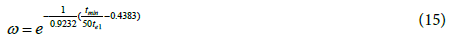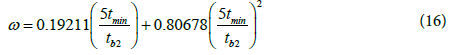Where te1 is the end time of the early infinite acting radial flow line, tb2 is the beginning time of the late infinite acting radial flow line and tmin is the minimum time.

3. The interporosity flow parameter: The interporosity flow parameter can be also obtained from the characteristic times as following: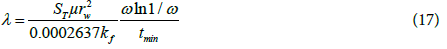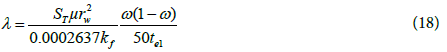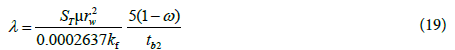Where ST is the product of the average bulk porosity (from cores or logs) and the average compressibility. λ can be also calculated from the minimum coordinates: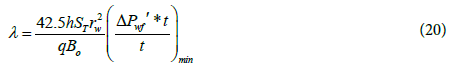In case ω less than 0.05, late transition period unit slope straight line is well observed. The interporosity flow parameter can be calculated from: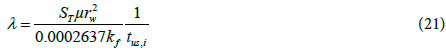Where tus,i the intersection of the transition period unit slope line with the infinite acting radial flow line.

4 Skin factor: The skin factor can be calculated from the early or late time radial flow pressure and pressure derivative data by using the following equations: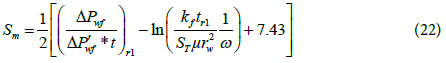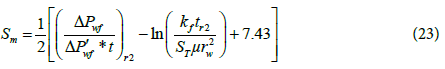Where r1 is any point on the early horizontal radial flow line and r2 is any point on the late horizontal radial flow line.

#### Infinite Acting Reservoir with Wellbore Storage

Wellbore storage effects can obscure early flow regimes on log-log plot of pressure and pressure derivative versus time. It is represented by early time unit slope straight line on the log-log plot. This unit slope period is followed by a peak on the pressure derivative curve as shown in Figure 2. The effect of wellbore storage can affect the minimum coordinates of the pressure derivative curve and cause the appearance of “pseudo-minimum” coordinates. Therefore, the effect of wellbore storage should be investigated prior to the analysis to know whether the observed minimum is the real minimum or the pseudo-minimum. For (tdw)min/(tdw)x ≥ 10, the wellbore storage doesn’t affect the minimum coordinates. [(tdw)x is the dimensionless time of the peak point, .

In case the minimum coordinates are not affected by wellbore storage, calculate the reservoir parameters using the following procedure :

1-Determine the fracture permeability using the late time radial flow line.

2-Calculate the wellbore storage coefficient from the early time unit slope using the following equations: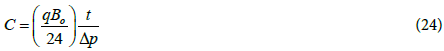Where t, Δp are any point on the unit slope line. (Δp=pi-pwf for drawdown and Δp=pws-pwf (Δt=0) for buildup tests)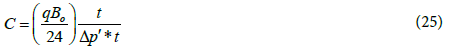The wellbore storage coefficient can also be calculated from the intersection time of the early time unit slope with the infinite acting radial flow line (ti).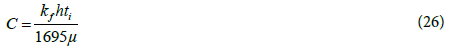3-Determine the ω and λ as outlined before.

4-Determine skin factor from the late time radial flow pressure and pressure derivative ratio.

If the minimum coordinates are influenced by wellbore storage, the interporosity flow parameter and the relative storativity can be calculated using the following equations:

Determine λ from the peak to minimum time ratio or from the peak to radial pressure derivative ratio: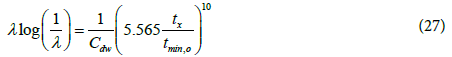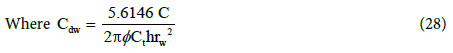(C: bbl/psi)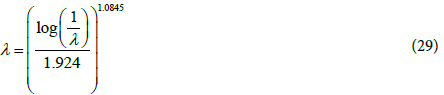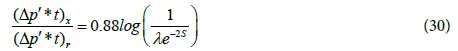Calcualte ω from the peak to beginning of second radial flow line time ratio: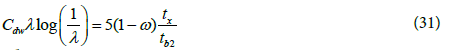Case 1

This case presents an oil field in Iran. A build-up test is conducted on a well from naturally fractured reservoir. The average core permeability received from the Iranian oil company ranges from 4 to 6 md. The well was flowing for 72 hours with q=2300 STB/day before shut-in for a build-up test. The build-up data are given in Table 1. The following reservoir and well data are also known:

h=280 ft tP=72 hrs

rw=0.281 ft Bo=1.35 bbl/STB

q=2300 STB/day μ=0.68 cp

Pwf (Δt=0)=2881 psia φ=0.15

Ct=1.5*10-5 psi-1

Δt, hr Pws, psia Δt, hr Pws, psia Δt, hr Pws, psia Δt, hr Pws, psia Δt, hr Pws, psia Δt, hr Pws, psia
0.0083 2881.36 0.6958 3232.181 3.5083 3393.13 49.6083 3489.319 53.7333 3493.64 57.85831 3497.62
0.0236 2901.811 0.7111 3235.39 3.6917 3395.58 49.7 3489.42 53.825 3493.73 58.0417 3497.82
0.0389 2916.59 0.7264 3238.541 3.875 3397.719 49.7917 3489.53 53.9167 3493.82 58.1333 3497.92
0.0542 2930.591 0.7417 3241.54 4.0583 3399.63 49.8833 3489.64 54.0083 3493.9 58.22501 3498.01
0.0694 2943.96 0.7569 3244.51 4.2417 3401.311 49.975 3489.73 54.1 3494 58.3167 3498.09
0.0847 2957.04 0.7722 3247.36 4.425 3402.97 50.0667 3489.83 54.1917 3494.09 58.40829 3498.18
0.1 2969.7 0.7875 3250.16 4.6083 3404.551 50.15829 3489.92 54.28329 3494.18 58.5 3498.26
0.1153 2981.93 0.8028 3252.86 4.7917 3406.05 50.25 3490.02 54.375 3494.28 58.59171 3498.35
0.1306 2993.64 0.8181 3255.5 4.975 3407.46 50.34171 3490.1 54.46671 3494.38 58.6833 3498.46
0.1458 3004.94 0.8333 3258.031 5.1583 3408.77 50.4333 3490.2 54.5583 3494.48 58.77499 3498.55
0.1611 3015.88 0.8486 3260.509 5.3417 3410.051 50.525 3490.3 54.65 3494.55 58.8667 3498.611
0.1764 3026.45 0.8639 3262.871 5.525 3411.27 50.6167 3490.39 54.7417 3494.66 58.9583 3498.68
0.1917 3036.69 0.8792 3265.21 5.7083 3412.42 50.7083 3490.5 54.8333 3494.729 59.05 3498.74
0.2069 3046.611 0.8944 3267.4 5.8917 3413.56 50.8 3490.61 54.925 3494.829 59.14169 3498.83
0.2222 3056.219 0.9097 3269.49 6.3417 3415.769 50.8917 3490.7 55.0167 3494.909 59.23331 3498.93
0.2375 3065.5 0.925 3271.529 7.2583 3420.139 50.9833 3490.8 55.1083 3494.99 59.325 3499.03
0.2528 3074.41 0.9403 3273.47 8.175 3423.5 51.075 3490.9 55.2 3495.06 59.4167 3499.13
0.2681 3083.03 0.9556 3275.35 9.0917 3426.319 51.1667 3490.99 55.2917 3495.13 59.5083 3499.22
0.2833 3091.33 0.9708 3277.17 10.0083 3428.771 51.2583 3491.07 55.3833 3495.24 59.60001 3499.32
0.2986 3099.31 0.9861 3278.931 11.8417 3432.46 51.35 3491.18 55.475 3495.35 59.6917 3499.4
0.3139 3106.99 1.0014 3280.63 13.675 3436.14 51.4417 3491.27 55.5667 3495.46 59.78329 3499.471
0.3292 3114.41 1.0583 3285.92 16.0083 3439.83 51.53329 3491.35 55.65829 3495.55 59.875 3499.579
0.3444 3121.581 1.15 3294.58 19.675 3446.42 51.625 3491.44 55.75 3495.65 59.96671 3499.669
0.3597 3128.46 1.2417 3302.33 23.3417 3453.72 51.71671 3491.53 55.84171 3495.74 60.14999 3499.859
0.375 3135.1 1.3333 3309.449 28.5083 3462.21 51.8083 3491.64 55.9333 3495.82 60.2417 3499.92
0.3903 3141.47 1.425 3316.091 34.0083 3470.219 51.9 3491.72 56.02499 3495.92 60.3333 3499.98
0.4056 3147.66 1.5167 3322.179 39.5083 3477.331 51.9917 3491.81 56.1167 3496.01 60.425 3500.049
0.4208 3153.619 1.6083 3327.91 45.0083 3483.611 52.0833 3491.93 56.2083 3496.09 60.51669 3500.149
0.4361 3159.34 1.7 3333.5 48.05 3487.63 52.175 3492.04 56.3 3496.18 60.60831 3500.25
0.4514 3164.89 1.7917 3338.809 48.1417 3487.72 52.2667 3492.12 56.39169 3496.241 60.7 3500.339
0.4667 3170.231 1.8833 3343.921 48.2333 3487.81 52.3583 3492.22 56.48331 3496.32 60.7917 3500.44
0.4819 3175.35 1.975 3349.42 48.325 3487.91 52.45 3492.32 56.575 3496.39 60.8833 3500.519
0.4972 3180.36 2.0667 3354.88 48.4167 3488.02 52.5417 3492.42 56.6667 3496.49 60.97501 3500.611
0.5125 3185.159 2.1583 3359.47 48.5083 3488.14 52.6333 3492.52 56.7583 3496.6 61.0667 3500.701
0.5278 3189.819 2.25 3363.501 48.6 3488.251 52.725 3492.59 56.85001 3496.69 61.15829 3500.77
0.5431 3194.3 2.3417 3367.15 48.6917 3488.34 52.8167 3492.66 56.9417 3496.78 61.25 3500.87
0.5583 3198.67 2.4333 3370.341 48.78329 3488.43 52.90829 3492.75 57.03329 3496.87 61.34171 3500.96
0.5736 3202.86 2.525 3373.25 48.875 3488.52 53 3492.85 57.125 3496.96 61.4333 3501.04
0.5889 3206.909 2.6167 3375.851 48.96671 3488.61 53.09171 3492.96 57.21671 3497.04 61.52499 3501.12
0.6042 3210.85 2.7083 3378.29 49.0583 3488.731 53.1833 3493.08 57.3083 3497.13 61.6167 3501.179
0.6194 3214.66 2.8 3380.48 49.15 3488.839 53.275 3493.18 57.39999 3497.22 61.7083 3501.24
0.6347 3218.36 2.8917 3382.509 49.2417 3488.929 53.3667 3493.26 57.4917 3497.32 61.8 3501.31
0.65 3221.94 2.9833 3384.409 49.3333 3489.029 53.4583 3493.36 57.5833 3497.4 61.89169 3501.39
0.6653 3225.449 3.1417 3387.269 49.425 3489.121 53.55 3493.45 57.675 3497.471 61.98331 3501.479
0.6806 3228.84 3.325 3390.44 49.5167 3489.219 53.6417 3493.56 57.76669 3497.55 62.1667 3501.659

Table 1: Pressure build up test Data for Case 1.

Semi-log analysis

Horner plot is shown in Figure 3. This figure depicts the early points that are affected by wellbore storage, however, the first straight line can be observed clearly. The figure shows two parallel straight lines that proves the dual porosity behavior. Therefore, the conventional semi-log analysis can be used to estimate reservoir parameters.

The fracture permeability can be calculated from the slope of the second straight line (m) to give: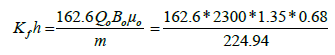=1526.23 md.ft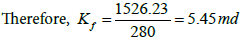The storativity ratio (ω) can be calculated from the vertical distance between the two straight lines (Δp) and the slope (m):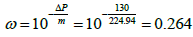A horizontal straight line through the middle of the transition region is drawn to intersect with the two semi-log straight lines. Read the corresponding times and calculate the interporosity flow coefficient (λ):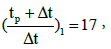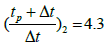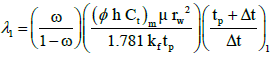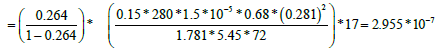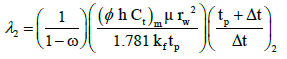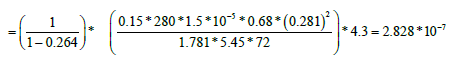#### Direct Synthesis Technique

The log-log plot of pressure and pressure derivative shown in Figure 4. It is clear that there is a wellbore storage with an early time unit slope and the early radial flow period is well defined. However, the late radial flow period not last for long time. The data exhibit a unique behavior which is indicative of a naturally fractured reservoir.

From Figure 4:

(t*ΔP?w)r2=99.7 psia tr2=32 hr,

ΔPr2=614 psia (t*ΔP?w)min=27.56 psia,

tmin=8.8 hr tus=0.054 hr,

ΔPus=49.6 psia tx=0.42 hr,

tb2=28 hr (t*ΔP?w)US=49.6 psia,

The effect of the WBS on the minimum derivative coordinates can be defined by calculating the ratio (tdw)min/(tdw)x tmin/tx=8.8/0.42=20.95 (>10).

Therefore the minimum derivative coordinates are the real minimum and not affected by wellbore storage.

The fracture permeability can be calculated from the late time infinite acting radial flow line: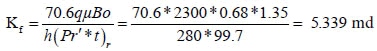Wellbore storage coefficient is calculated by: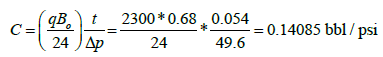Skin factor from the late time pressure and pressure derivative data: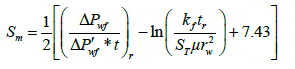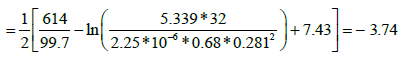The dimensionless storage coefficient (ω):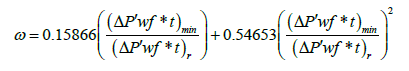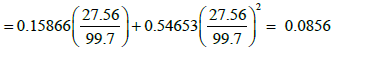The interporosity flow parameter (λ):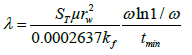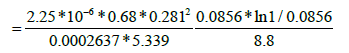=2.051 * 10-6

For verification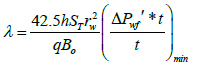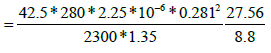=2.132 * 10-6

Comparison of the results of conventional semi-log analysis, direct synthesis technique, and type curve matching is shown in Table 2. The results of the semi-log analysis are only matching with the direct synthesis and software results in permeability. However, the storage coefficient and the interporosity flow parameter are inaccurate. On the other side, the direct synthesis technique and the software results show an excellent match in all reservoir parameters.

Parameter Conventional semi-log Direct synthesis Software matching
Kf (md) 5.45 5.339 5.375
ω 0.264 0.0856 0.0865
λ 2.955*10-7 -2.828*10-7 2.051*10-6 - 2.132*10-6 2.012*10-6
S   - 3.74 -3.71
C (bbl/psi)   0.14085 0.1453

Table 2: Comparison of the Results of the Case 1.

Naturally fractured reservoirs with a vertical fracture

The pressure behavior of a dual porosity formation intersected by uniform flux and infinite conductivity fracture can be investigated using log–log plots of pressure and pressure derivative functions. The direct synthesis technique can be used to calculate reservoir parameters such as skin, wellbore storage, permeability, interporosity flow parameter, relative storativity and half-fracture length without type curve matching. The applied assumptions are: the reservoir is isotropic, horizontal, and has constant thickness and fracture permeability. The fractured well is producing at constant rate with constant viscosity, slightly compressible fluid. In addition, the fracture fully penetrates the vertical extent of the formation and has the same length in both sides of the well. A pseudosteady state interporosity flow between the matrix and the fracture system is also assumed.

#### Uniform Flux Fracture

Figure 5 shows the pressure derivative plots for various values of Xe/ Xf ratios, in a single layer square, dual porosity reservoir with pseudosteady state interporosity flow. Three flow regimes are shown in these figures: the linear flow regime, infinite acting radial flow regime, and pseudosteady steady state flow regime .

Figure 5: Pressure derivative response in a single-layer square, naturally fractured reservoir with pseudo steady state inter porosity flow. Both WBS and Skin are ignored.

1) Linear flow period: The linear flow period occurs at early time. During this period, the flow resulted from the expansion of the fluid within the fracture network as the matrix effect is negligible. The linear flow period can be identified by a straight line of slope 0.5. This straight line is used to calculate the fracture half length.

The equation of pressure derivative during this flow regime is: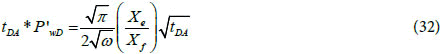By taking logarithm of both sides of the equation gives: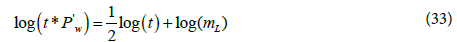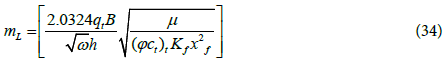Based on Eq. (34) the log-log plot of pressure derivative versus time gives half slope straight line during the linear flow period. The fracture half-length can be calculated by: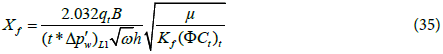where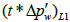is the value of pressure derivative at t=1hr on the linear flow line.

2) Pseudoradial flow period: The infinite acting radial flow period is dominated only for (Xe/Xf) > 8, as shown in Figure 5. This flow regime is identified by a horizontal straight line on the pressure derivative plot and can be used to calculate permeability and skin .

The pressure derivative equation during this flow regime is: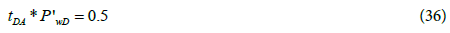The above equation in dimensional from yields: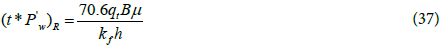R stands for radial flow. Solving the above equation for permeability gives: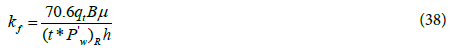The skin can be determined by: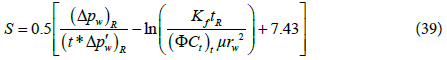3) Pseudosteady state flow period: In case of a vertically fractured well inside a closed system, a third straight line of unit slope appears. This line corresponds to the pseudosteady state flow regime is used to calculate the drainage area and shape factor.

The pressure derivative equation describing this flow period is: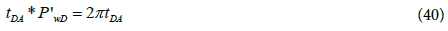By taking logarithm of both sides of the above equation, the dimensional form is: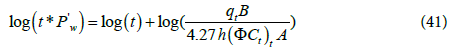By substituting t=1hr, the drainage area can be calculated using the following equation: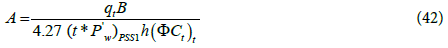Where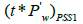stands for pseudosteady state flow period at time equal 1 hr.

The shape factor, CA, can be calculated by the following equation: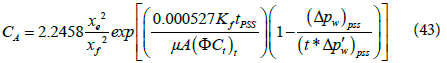4) Transition period: The transition can occur during the infinite acting radial flow as shown in Figure 5. In this case, the relative storativity, ω, and the interporosity flow parameter, λ, can be estimated by several methods as previously described in the previous section. If the transition takes place during the linear flow period as shown in Figure 6, two parallel straight lines of slope equal 0.5 can be observed. The first line represents the expansion of the fracture network, this flow period is called “fracture storage dominated flow period”. While the second line appears during the total system dominated flow period (for this period ω=1). Also, a straight line of unit slope is observed during late transition period. The intersection time of the straight lines of different flow regimes have been used in several equations to calculate reservoir parameters in case one of the flow regimes is missing or for verification purposes. These equations are presented in the following procedure:

Figure 6:Pressure derivative response in a vertical fractured reservoir with pseudo steady state inter porosity flow. The transition occurs during the linear flow period.

Step 1 - Plot the pressure difference ΔP and the pressure derivative (t*ΔP?w) versus time on log-log plot and identify different flow regimes.

Step 2 - Calculate the fracture permeability from Eq. (38).

Step 3 - Calculate ω and λ as outlined before.

Step 4 - If the transition occur during linear flow regime and the two parallel straight lines of slope 0.5 observed, verify ω using the following two equations: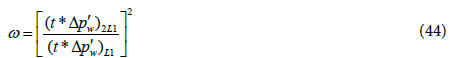where 2L1 stands for the linear flow at the total system dominated regime, and L1 stands for fracture storage dominated flow regime.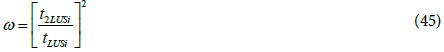where t2LUSi stands for the intersection point between the late transition period unit slope line and total system dominated flow line, and tLUSi stands for the intersection point between the late transition period unit slope line and the fracture storage dominated flow period.

Step 5 - Read the value of (t*Δp?w) at time 1 hr from the linear flow line (extrapolated if necessary), (t*Δp?w)L1.

Step 6 - Calculate the fracture half-length, Xf, from the linear flow straight line (Eq. 35).

If the linear flow not observed (due to wellbore storage or noise), then fracture half-length can be calculated from the half slope pressure Δpw instead of pressure derivative as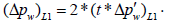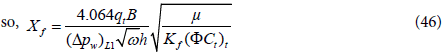then draw a straight line of slope 0.5 parallel to the pressure straight line to cross the (t*Δp?w)L1.

Step 7 - Determine the intersection between the linear and radial flow line tLRi from the log- log plot of the derivative (t*Δp?w) curve.

Step 8 - Calculate the ratio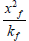from equation: (for square geometry A=xe2)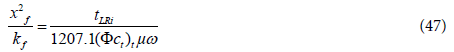Compare this ratio with the previously calculated values of Xf and Kf. If the two ratios are nearly equal, then the values are correct. If they are different, shift one or both straight lines then repeat the previous steps until their values approach.

Step 9 - Determine the value of (t*Δp?w)PSS1 from the pseudosteady state line and find the drainage area A from: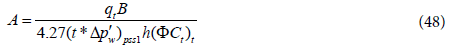Step 10 - Read the intersection time of the infinite acting line and the pseudosteady state line (tRPSSi) from the plot and calculate the drainage area A: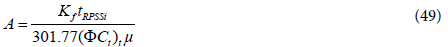Areas from steps 10 and 11 should be equal. If they are not equal, shift the lines left or right and repeat the calculations.

Step 11 - Determine the interporosity flow parameter after stimulation (λf) from: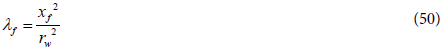Step 12 - Verify (λf) using the late transition period unit slope line by the following equations: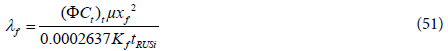where tRUSi stands for the intersection time between the late transition period unit slope line and the infinite acting line.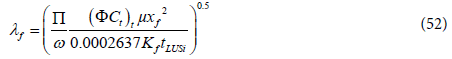where tLUSi stands for the intersection time between the late transition period unit slope line and the fracture storage dominated linear flow period.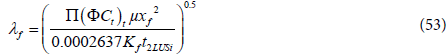where 2LUSi stands for the intersection point between the late transition period unit slope line with the total system dominated linear flow period.

Step 13 - Calculate skin using Eq. (39).

Step 14 - Calculate the shape factor from the value of Δpw and (t*Δp?w) corresponding to any convenient time during the pseudosteady state flow regime using Eq. (43).

#### Infinite Conductivity Fracture

The pressure and pressure derivative obtained for infinite conductivity fracture are the same as the uniform flux fracture except for a fourth dominated flow regime called bi-radial flow. This flow regime can be identified by a straight line of slope 0.36. It corresponds to the transition period between the early time linear flow regime and the infinite acting radial flow regime4. The characteristics of the linear, radial, and pseudosteady state flow periods are the same as illustrated earlier in the case of uniform flux fracture. The characteristics of the bi-radial flow regime are as following:

The bi-radial flow regime can be identified from the pressure derivative function by a straight line of slope 0.36. However, it cannot be identified from the pressure function. In case the linear flow line is not observed, the bi-radial flow line is used to determine the fracture half length. The pressure derivative equation describing the bi-radial flow period is :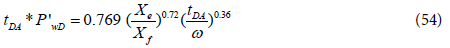By taking logarithm of both sides of the above equation, the dimensional form is: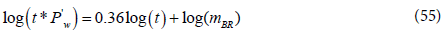where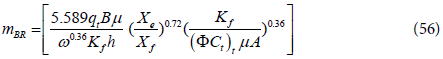Solving for the fracture half-length, Xf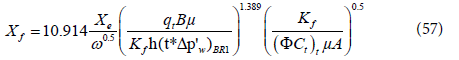If all the flow regimes that were found in the case of uniform flux fracture are available, use the previous procedure of the uniform flux fracture to analyze the pressure test for infinite conductivity fracture.

However, in case the linear flow line is either too short or not observed, the following procedure4 can be used:

Step 1 - Plot the pressure difference ΔP and the pressure derivative (t*ΔP?w) versus time on log-log plot and identify different flow regimes.

Step 2 - Determine the value of (t*ΔP?w)R from the infinite-acting radial flow line.

Step 3 - Determine the fracture permeability as discussed before in uniform flux fracture.

Step 4 - Calculate ω and λ as outlined before.

Step 5 - Verify ω as discussed before in the case of the uniform flux fracture.

Step 6 - Read the value of (t*Δp?w)PSS1 corresponding to the pseudosteady state line and determine the drainage area (A).

Step 7 - Read the intersection time of the infinite acting line and the pseudosteady state line (tRPSSi) from the plot to determine A (Eq. 49).

Areas from step 6 and 7 should be equal, if not shift left or right and repeat the steps.

Step 8 - Read the value of (t*Δp?w) at time t=1hr from the bi-radial flow line, (t*Δp?w)BR1. The bi-radial flow line can be extrapolated if necessary.

Step 9 - Determine the fracture half length, Xf, from Eq. (57)

Step 10 - Calculate the ratio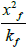using the values of step 3 and 9.

Step 11 - From the plot, read the time of intersection of the radial flow and the bi-radial flow line, tRBRi, then determine the ratio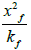from4: (for square geometry Xe=Ye)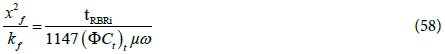If Step 10 and 11 are the same, then Xf and Kf are correct and if they not the same, shift one or both lines (bi-radial and infinite acting) and repeat all the procedure.

Step 12 - Determine the interporosity flow coefficient after stimulation (λf) by Eq. (50).

Step 13 - Verify (λf) as previously outlines.

Step 14 - Calculate the skin factor by Eq. (39).

Step 15 - Calculate the shape factor from the value of Δpw and (t*Δp?w) corresponding to any convenient time during the pseudosteady state flow period by Eq. (43).

Case 2

Britt et al.  interpreted the pressure fall off test performed on a well. The well has been acidized several times before fracture stimulating in November 1982. The test was performed using down hole shut-in device and pressure gauges. The pressure fall off test data are shown in Table 3 and the following reservoir and fluid data are known:

Δt, hr Pws, psia Δt, hr Pws, psia
0 1183 0.066358 1127.6
0.000556 1171.7 0.083285 1121.6
0.00111 1170.4 0.38287 1073
0.001667 1169.4 0.71312 1060
0.002222 1168.6 0.87794 1049.9
0.0025 1168.3 1.0424 1041.7
0.003055 1167.4 1.3701 1034.7
0.003611 1166.6 1.859 1023.4
0.004167 1166 2.3445 1010.3
0.004722 1165.2 2.8267 1000
0.005278 1164.6 3.3056 991.6
0.005555 1164.2 3.7813 984.4
0.006111 1163.7 4.2538 978.4
0.006667 1163 5.1893 972.7
0.0075 1162 6.1123 963.1
0.0083 1161.3 12.87 955
0.01527 1155.1 19.559 915.4
0.016668 1154 23.884 892.7
0.019997 1151.6 27.265 881
0.022217 1150 29.204 873.1
0.033325 1143.2 31.079 868.7
0.049703 1134.7 33.485 859.5

Table 3: Pressure fall off test data of Case 2.

h=135 ft Ct=9.5*10-6 psi-1 rw=0.25 ft Bo=1 resbbl/STB

q=1050 bbl/day μ=0.7 cp

K=3.33 md φ=0.085

Figure 7 shows the log-log plot of pressure and pressure derivative versus time. It is clear from the log-log plot that the transition period occurs early during the linear flow regime. Consequently, two parallel straight lines of half-slope appear. The first line resulted from the expansion of the fracture network, while the second line represents the total system behavior. Also, the infinite acting radial flow line is detected but not long enough. The pressure derivative plot exhibit a unique behavior of a hydraulically fractured well in a naturally fractured reservoir. Britt et al.  analyzed the pressure behavior of this well by using the type curves of homogeneous reservoirs with hydraulic fracture. Therefore, they cannot estimate the values of the relative storativity and the interporosity flow coefficient.

From Figure 7:

(t*ΔP?w)r=110 psia tr=23 hr

ΔPr=290 psia (t*ΔP?w)2L1=21 psia

(t*ΔP?w)L1=100 psia

Direct Synthesis technique is used to estimate the reservoir parameters as following:

The fracture permeability can be calculated from the infinite acting radial flow line: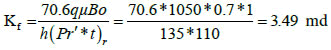The relative storativity can be calculated from the two parallel half slope straight lines: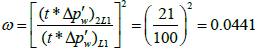Calculate the fracture half-length from the linear flow straight line: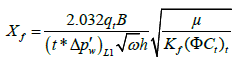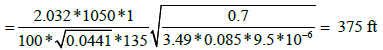The fracture half-length can also be calculated from the total system dominated flow period: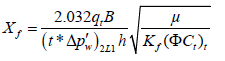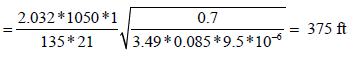The interporosity flow parameter after stimulation (λf) can be estimated from the original interporosity flow parameter: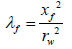Where,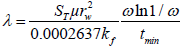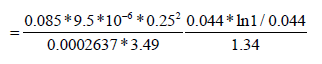=5.62*10-6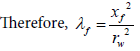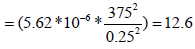Calculate skin by reading any convenient point during the infinite acting period: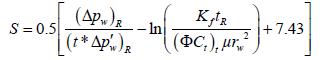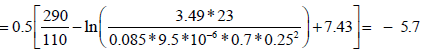The differences between the results of the type curve matching obtained by Britt et al.  and the results of direct synthesis technique are shown in Table 4. The fracture permeability from type curve matching nearly the same as that from direct synthesis, while the fracture half lengths are very close.

Parameter Type curve matching by Britt et al. Direct synthesis technique
Kf, md 3.33 3.49
Xf, ft 442 375
ω   0.0441
λ   5.62*10-6
λf   12.6
Skin   -5.7

Table 4: Comparison of Results of Case 2.

#### Conclusions

1. The use of pressure derivative plots improved the analysis of well test data. Different flow regimes can be identified on the derivative log-log plots. Type curve matching can give good results in case all of the flow regimes are identified.

2. In this study, Tiab direct synthesis technique was shown to be accurate and simple. It gave direct estimates of reservoir parameters and fracture characteristics by using a log-log plot of pressure and pressure derivative data without type curve matching.

3. In case of high wellbore storage, the conventional semi-log analysis gives inaccurate results and cannot estimate all naturally fractured reservoir parameters.

4. When not all the flow regimes are identified, type curve matching gives non-unique solution. However, the direct synthesis technique gives accurate results of the naturally fractured reservoir parameters and fracture properties.

5. The direct synthesis method, showed accurate results compared to commercial software matching. It can be used to calculate the reservoir and fracture properties in case of a well crossed by a uniform flux or infinite conductivity fracture.

6. In case of naturally fractured reservoirs with a vertical fracture, if the transition period occurs during the linear flow, two parallel straight lines of slope 0.5 appear on the pressure derivative plot. This pressure derivative behavior can be used in calculating different reservoir parameters.

--

### Article Usage

• Total views: 5619
• [From(publication date):
December-2016 - Sep 23, 2023]
• Breakdown by view type
• HTML page views : 4598Can't read the image? click here to refresh